# VBA Set RangeArticle byExcelMojo Team## What is Set Range in Excel VBA?

VBA Set Range helps us set a range in a single cell, multiple cells, rows, or columns anywhere in the Excel sheet, where we must perform some tasks using the VBA code. If we do not use this function, then the current cell, where the cursor is placed, is automatically chosen.

Let us understand the use of VBA Set Range with a simple example.

Write the below code in VB editor to choose the range of cells for displaying the value “Hello.”

Run the code by clicking the “Run” option in the VB ribbon. We can see ”Hello” is placed in cells A2 to B4.

###### Key Takeaways
• VBA Set range allows us to choose multiple cells to insert our desired value.
• When using the Cell property to access a cell, we must use a row and a column combination as a cell address. We can also set the range reference by declaring a VBA set range variable.
• The syntax for Range in VBA is shown below:
• Here, Cell1 and Cell are the cell address of rows or columns.

### How to Access Range of Cells in Excel VBA?

We can access a range in Excel VBA in two ways:

1. Directly using Range Object
2. Using the VBA Cells property

Let us see an example to access cell A1 using the Range object and the Cells property.

1. Open the Excel workbook and click on the “Developer” option. Select “Visual Basic” to open the VB Editor. In the “Insert” option, choose “Module” to open a new module.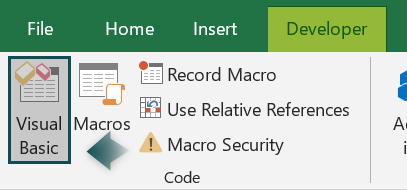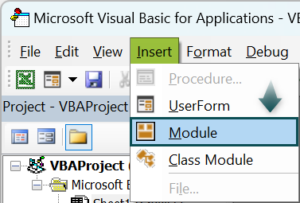2. Start writing the sub-procedure, opening the Range object, and entering the cell address in “double quotation.”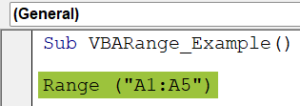3. After this, put a . dot, and you will see the properties and methods of the Range object. For example, as we wish to add a value to the cell, we will select the “Value” property.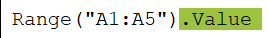4. Use an equal to sign = and write the value you wish to see in the selected cells. Here, we write, “Welcome to India.”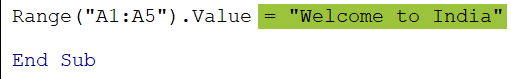Sub VBARange_Example()
Range(“A1:A5”).Value = “Welcome to India”
End Sub

5. Run the code using the Run option in the VB ribbon or pressing F5, and you will see the value inserted into the desired cells (A1-A5).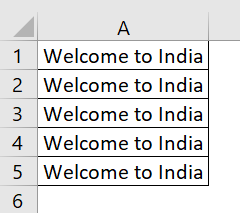Likewise, we can also use the “Cells” property. Here, we must give a row and column number as cell addresses. We can access only one cell to insert our value in this option.

• Step 1: Write the sub-procedure and declare the Cells property and mention a row and a column number as shown below:

Sub CELLS_Examples()
Cells(1, 2).Value = “Data is Significant”
End Sub

Here, Cells (1, 2) means Row 1 and Column 2, i.e., B1 cell

• Step 2: Use the “Value” function to write the value in “ double quotation.”
• Step 3: Run the code. We can see the value inserted into the desired cell B1, as shown below.

### Accessing Multiple Cells & Setting Range Reference in VBA

This section will see how to access multiple cells using the Range object in VBA. For example, suppose we want to place our value in multiple cells ranging from A1-A10 and C1-C10 instead of one cell. Here, we can use the Range Statement.

Below are the steps to access multiple cells using Range object:

• Step 1: Write the code starting with the Sub procedure and declare “Rng” as Range. So that we don’t need to write Range always; instead, only Rng is used.
• Step 2: Now, we will set the reference range using the “SET” keyword and write the cell address using the Range object.
• Step 3: Now, assign the value to the cells from the range A1-A10.
• Step 4: Similarly, we will set another range of cells C1-C10 and assign a value as shown below:

Sub Range_Examples()
Dim Rng As Range
Set Rng = Range(“A1:A10”)
Rng.Value = “Result is 100%”
Set Rng = Range(“C1:C10”)
Rng.Value = “Result is 50%”
End Sub

• Step 5: Run the code, and we will see the assigned value is inserted in our selected range of cells (A1-A10 and C1-C10).

### Important Things to Note

• VBA Set Range is used to select multiple cells, while VBA Set Range with “Cells” is used to access one cell only.
• VBA Set Range is an Object, whereas Cells is a property.
• VBA Set Range value can be inserted into multiple combinations within the cells in the Excel workbook.
• It is very simple to insert multiple values in cells using Range function in VBA.
• We can also make changes to the existing cell format using the Range object.
• To refer to a range of cells. There are three different ways available:
• Range Object
• Cells Property
• Offset Property
• We can also select a 3D range of cells in Excel using the Range object in VBA.
• Offset property is used to refer to a cell that is some number of rows or columns away from the desired cell address.

1. How do I set a range to an array in VBA?

To VBA set range to array, we need must follow the following steps:
1) Declare a dynamic array using the variant data type.
2) Declare another variable for storing the count of cells from the range and use that count for the loop too.
3) Assign the range where we have value to the array.
4) Create a loop to print all the values in the current window, and you can see all the assigned values to the array.

2. Can you set a range as a variable in VBA?

Yes, Set statements are used to set a range to a variable in VBA. For this, we first declare the variable of range data type and then specify a range of cells using the Range object. It will further allow us to use all the relative properties and methods of the Range object.

3. How do I set an active range in VBA?

To set an active range in VBA, follow the following steps:
Open an Excel worksheet.
Press Alt+F11 or select the Developer option to Open VBA Editor
Insert a Module from the Insert option
Write the code for activating a range in VBA Editor
Save the file as a macro-enabled workbook.
Press ‘F5’ to run it or ‘F8’ to debug the code line by line.

4. What keyword can a range be set using in VBA?

The “Set” keyword sets a range of cells in VBA. It remains fixed throughout the code in VBA.